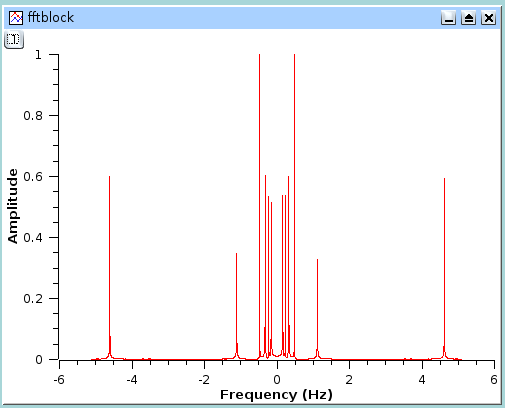# Filtering of data curves

In this section, it will be assumed that you have the following data curve: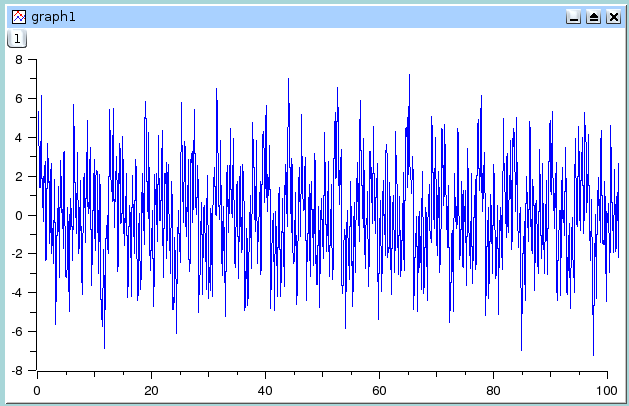This signal has a power spectrum which contains both high and low frequencies. We can analyze this by doing a FFT on the data curve, this leads to the following figure:The next sections will show the influence of the different filters on this data curve.

## FFT low pass filter

This filter cuts high frequencies from a signal. You just have to select the cut-off frequency of the filter. Let us assume that we want to keep the frequencies below 1 Hz, we will obtain:

Figure 6-11. Signal after a FFT low pass filter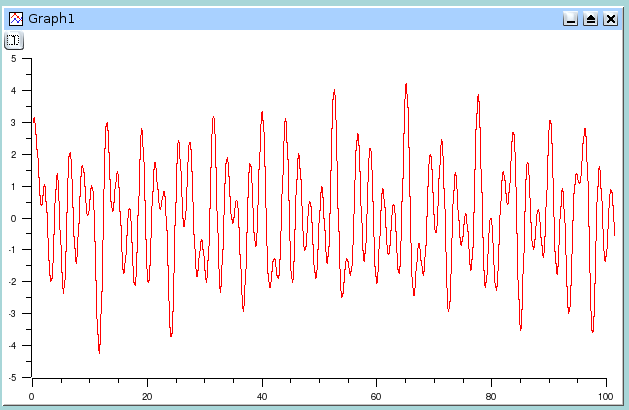The power spectrum of this new signal shows that the frequencies below 1 Hz.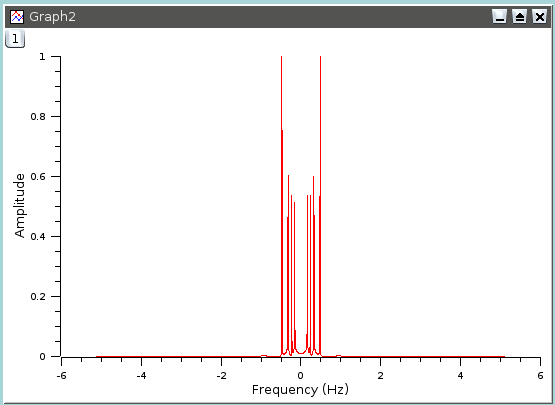## FFT high pass filter

This filter cuts low frequencies from a signal. You just have to select the cut-off frequency of the filter. Let us assume that we want to keep the frequencies above 1 Hz, we will obtain:

Figure 6-12. Signal after a FFT high pass filterThe power spectrum of this new signal shows that the frequencies above 1 Hz remain.## FFT band pass filter

This filter cuts both low and high frequencies from a signal while leaving frequencies in the pass band. You just have to select the high and low cut-off frequencies of the filter. Let us assume that we want to keep the frequencies between 1.5 and 3.5 Hz, we will obtain:

Figure 6-13. Signal after a FFT band pass filter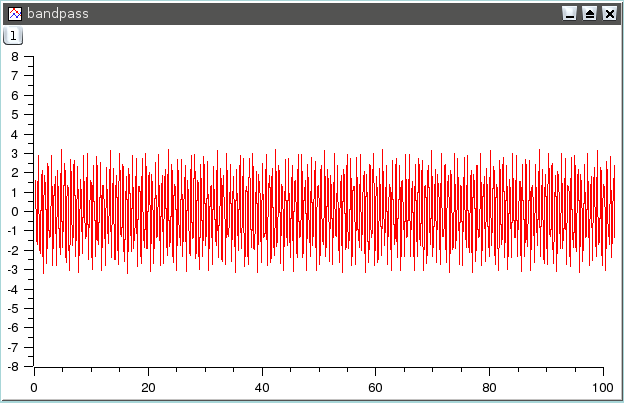The power spectrum of this new signal shows that only frequencies in the pass band remain (2 and 3 Hz).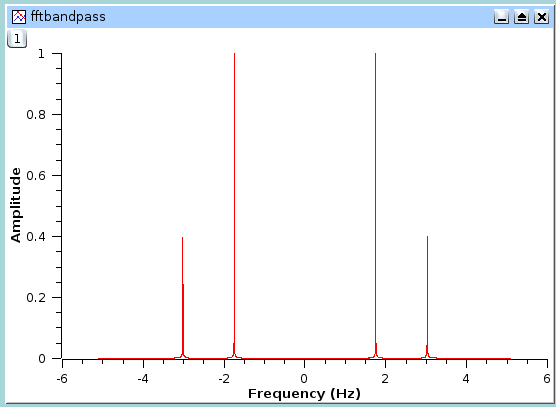## FFT block band filter

This filter keeps the low and high frequencies in a signal while cutting frequencies in the stop band. You just have to select the high and low cut-off frequencies of the filter. Let us assume that we want to remove the frequencies between 1.5 and 3.5 Hz, we will obtain:

Figure 6-14. Signal after a FFT block band filter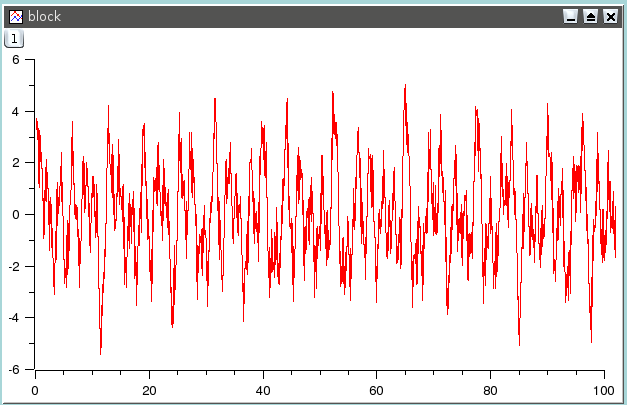The power spectrum of this new signal shows that only the frequencies below 1.5 Hz and above 3.5 Hz remain.